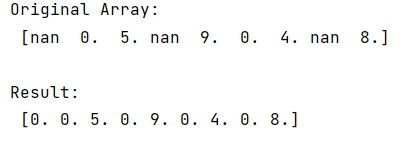# Convert nan value to zero in NumPy array

Learn, how to convert nan value to zero in NumPy array?
Submitted by Pranit Sharma, on January 07, 2023

NumPy is an abbreviated form of Numerical Python. It is used for different types of scientific operations in python. Numpy is a vast library in python which is used for almost every kind of scientific or mathematical operation. It is itself an array which is a collection of various methods and functions for processing the arrays.

## Converting nan value to zero

While creating a DataFrame or importing a CSV file, there could be some NaN values in the cells. NaN values mean "Not a Number" which generally means that there are some missing values in the cell.

Suppose we are given a NumPy array with some nan values and we want to replace these nan values with zeroes.

For this purpose, we will use the isnan() method which produces a bool array indicating where the NaN values are. A boolean array can be used to index an array of the same shape. We will use it as a mask.

Let us understand with the help of an example,

## Python code to convert nan value to zero in NumPy array

```# Import numpy
import numpy as np

# Creating an array
arr = np.array([np.nan,0,5,np.nan,9,0,4,np.nan,8])

# Display original array
print("Original Array:\n",arr,"\n")

# Assigning 0 in place of nan values
arr[np.isnan(arr)] = 0

# Display result
print("Result:\n",arr,"\n")
```

Output: